|

# 90后情侣爆改50平米旧屋!生活永远不是租来的

过自己的小日子

让别人羡慕得跺脚吧想想和大叔

毕业季那年

两人纷纷选择在北京落脚

找到心仪的工作后

在两家公司中间的区域也找到了新租屋

一间只有50㎡的大开间

一厨一卫，不分客厅卧室

朝北的窗台带有落地窗

只是一到冬天，墙壁必定发霉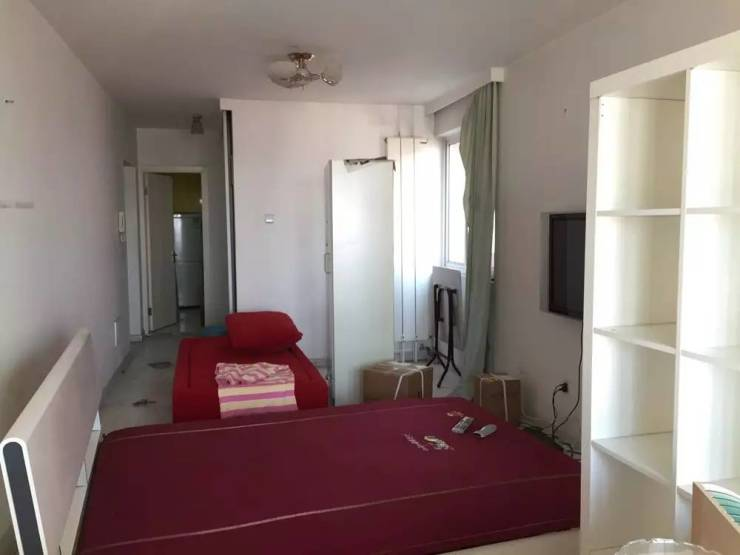两年里，他们经历过煤气泄漏

管道老化、水漫金山

也度过2次难忘的圣诞节

养活12盆生命力旺盛的植物

煮了39顿热腾腾的火锅

烘焙了200多个甜点

看过300多部电影两年前走进租屋的第一眼感觉：旧！

装修粗糙、家具破旧

厚重的绿色窗帘

抖一抖全是积灰

墙体发黄倒还算好的

有些损坏严重的地方已经完全剥落了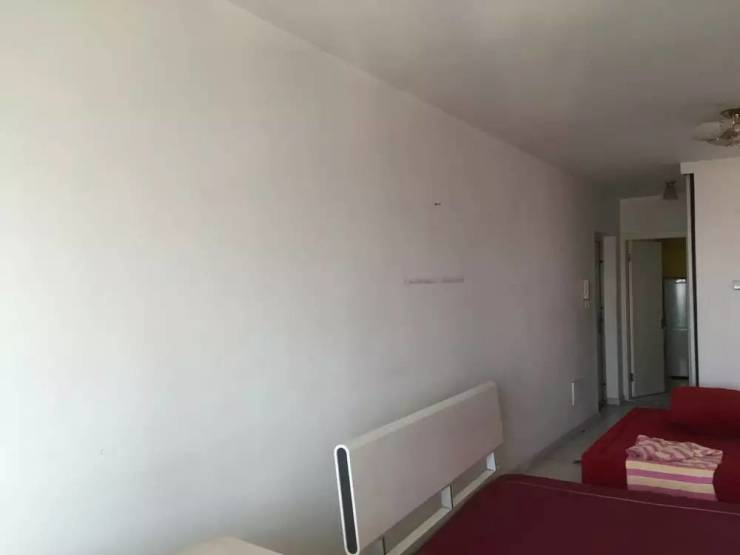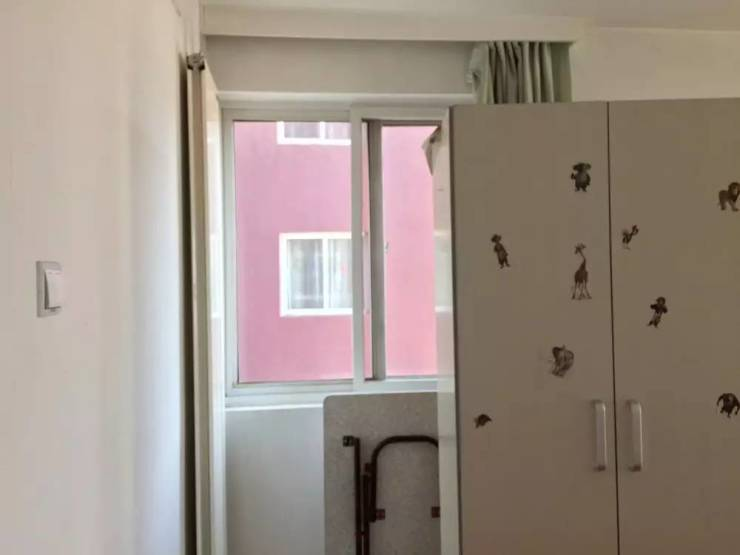厨房向来是油烟的“重灾区”

橱柜、水槽、料理台、瓷砖上都积了一层厚重的油垢

发黑长毛到房君都不忍形容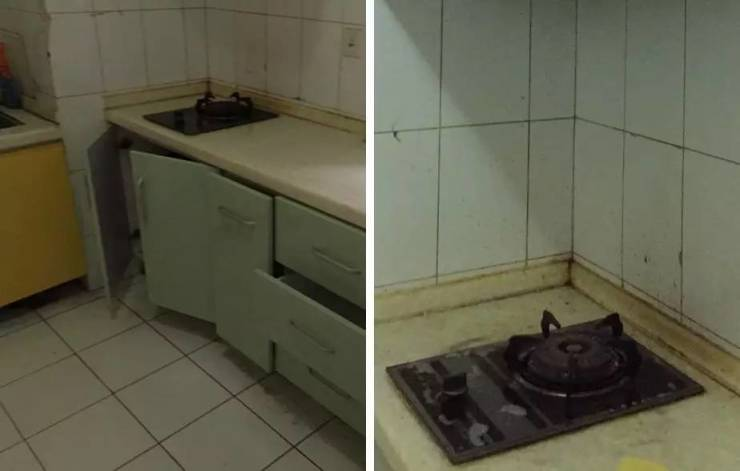因为没有客厅和卧室的空间区分

原屋主将床和沙发靠在一起

电视机在床尾

饭桌在窗前

相当于将客厅、餐厅、卧室融为一体

由于规划无序

整个大开间显得无比局促不过好在50㎡的居住面积

对于两个人来说，是足够了的

于是，在征得房东同意后

想想和大叔的改造计划开始了

第一步从刷漆开始

从网上买来环保漆、短毛油漆滚筒和必备的伸缩杆

再找两件旧外套

就开始调漆

没有经验的小白

调出来的漆不够浓稠

每面墙都至少刷了3、4次

而对于这间屋子的归属感

一切都从亲力亲为开始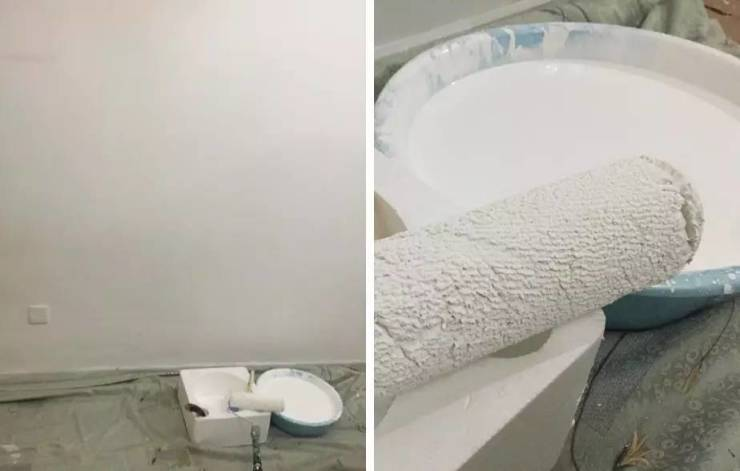焕然一新的大白墙白净清爽

搭配各种墙饰

空间感十足颜色清淡

线条简单

整个空间都变轻盈了原先的红色旧沙发

落满了毛发和碎屑

清洗起来工作量实在太大

决定换个北欧风的灰色沙发

PS：上镜的小细腿最能拯救小户型的拥挤感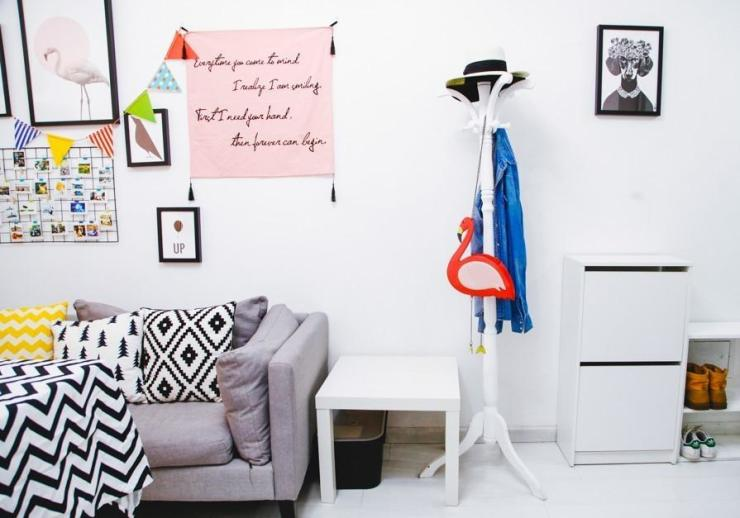沙发前好不容易留出的宽敞空间

是想想的健身专有地

一周三次

逐渐形成的马甲线令她元气十足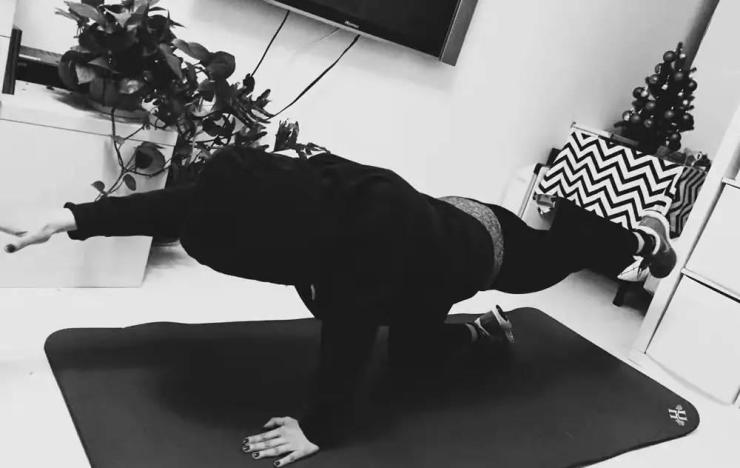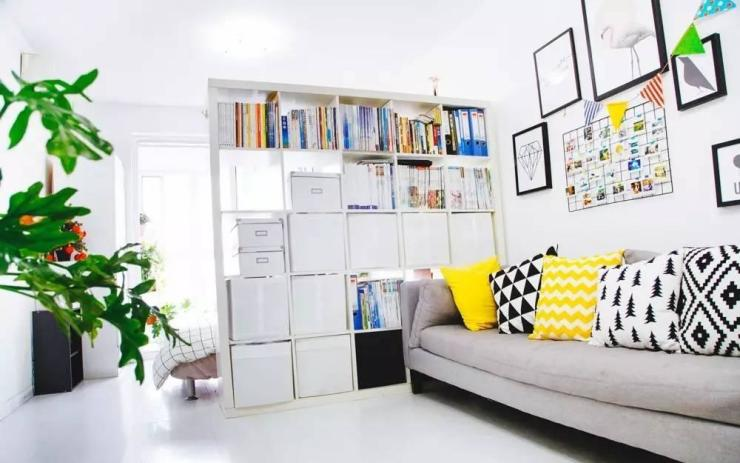吸取了前屋主的经验

开间的规划一定得明晰

一个5X5的大书架横在沙发和床之间

同时解决了收纳和隔断的问题

又保证了卧室的私密性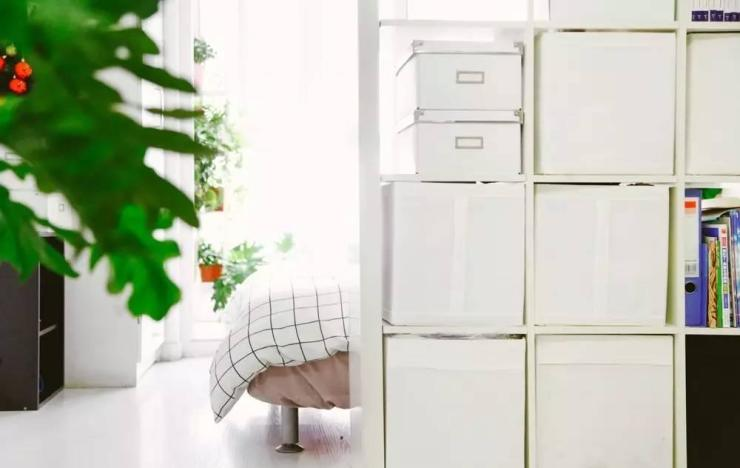而它也是最好的“床头柜”

不用起身即可取下睡前书本

与阳台相通的玻璃移门

让人每天早上都能感受到阳光的温度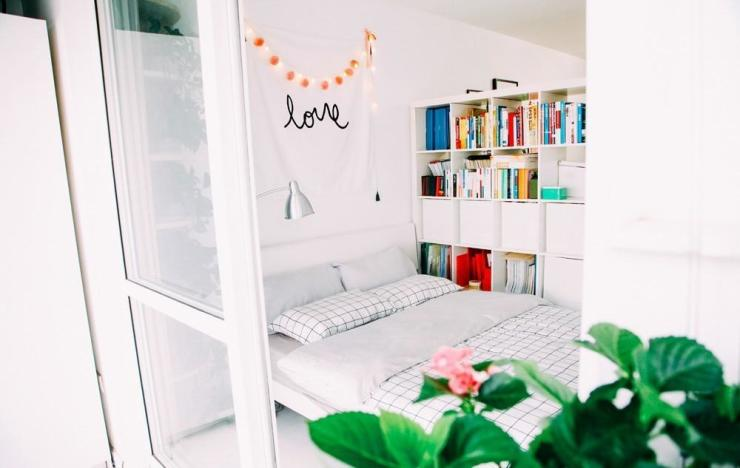拆了旧窗帘

换成定制的白色纱帘

光能透进来，风也能吹起来一株绣球、两颗春羽、三盆薄荷

阳台就是花草的世界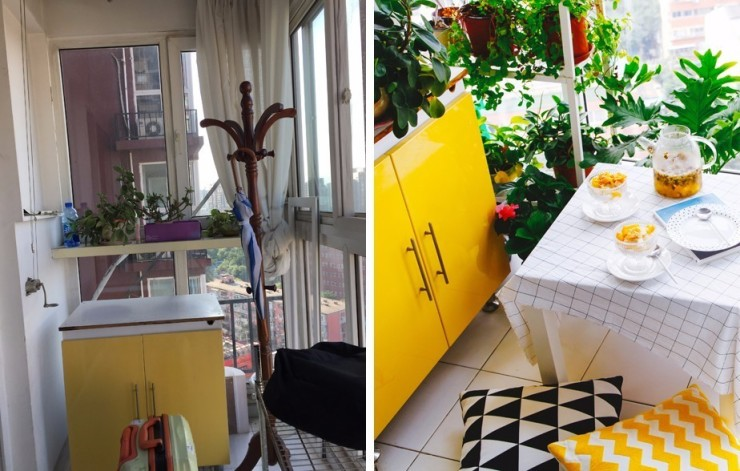一张小桌、两把座椅

一块蛋糕、两杯冷泡茶

如此惬意又鲜活的午后

在每个闲暇的周末，都会在阳台上演悄然盛开的绣球花

偶尔飞过天空的鸽子

天气晴朗时还能望见西山

小小的一方阳台装下了无数对生活的眷恋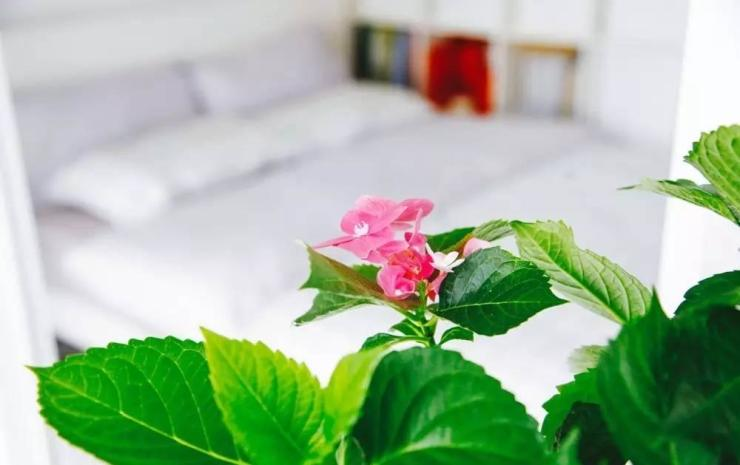房东留下了不少老家具

虽然都有些年代感

但没什么问题是刷漆解决不了的

两个柜子磨损得厉害

颜色也有些发黄

粉刷一层

宛若两个崭新的柜子酒红色的木质衣帽架

走的是当下流行的老干部风

但也和想想追求的小清新风不太搭

用油漆刷成白色

似乎年轻了一个世纪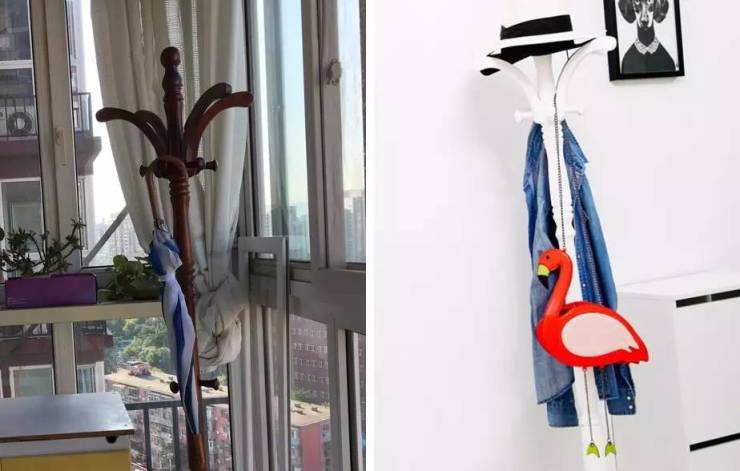厨房的打理最耗时

油垢最顽固

用钢丝刷和重油污清洁剂

奋斗了一个下午

才让原本脏黑的瓷砖闪闪发亮灯泡都换成节能灯

装了一个新油烟机

经过“整容”后的厨房宛若新生

对于爱做饭的想想和大叔来说

这便是最好的乔迁之礼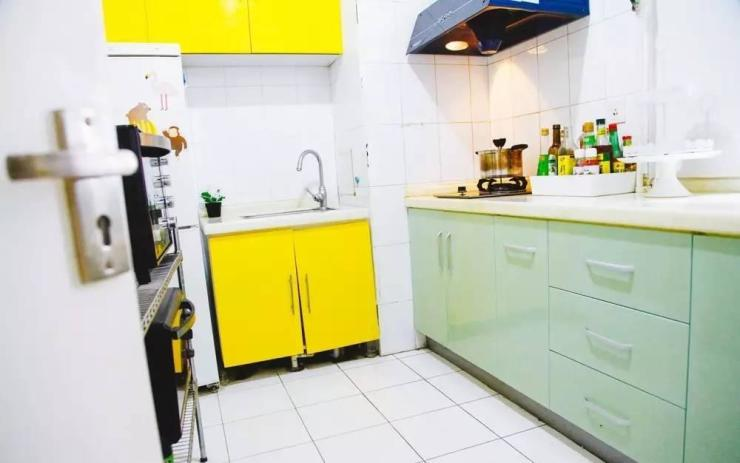种类丰富的香料和五谷杂粮

分装在密封性很好的储物盒里

背面写上保质期

爱做饭的人也爱整理做饭的地方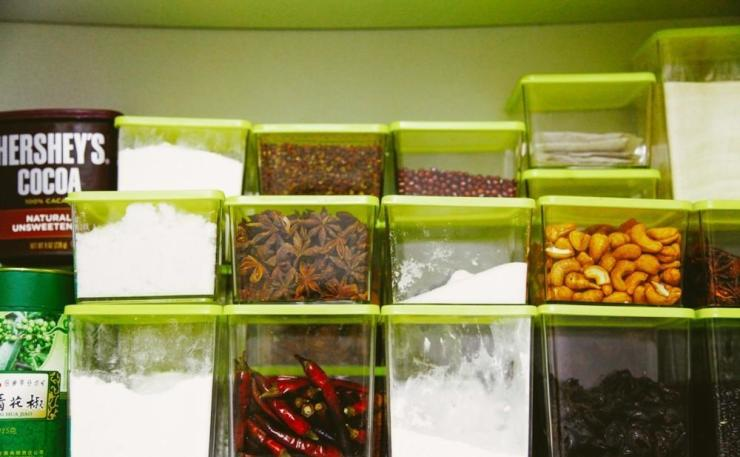刀具、叉子、勺子在收纳盒里各归其位

分类得清清楚楚、一目了然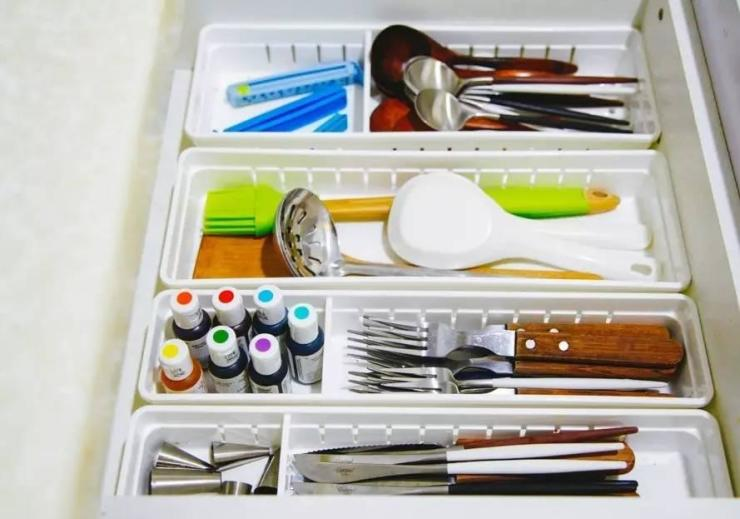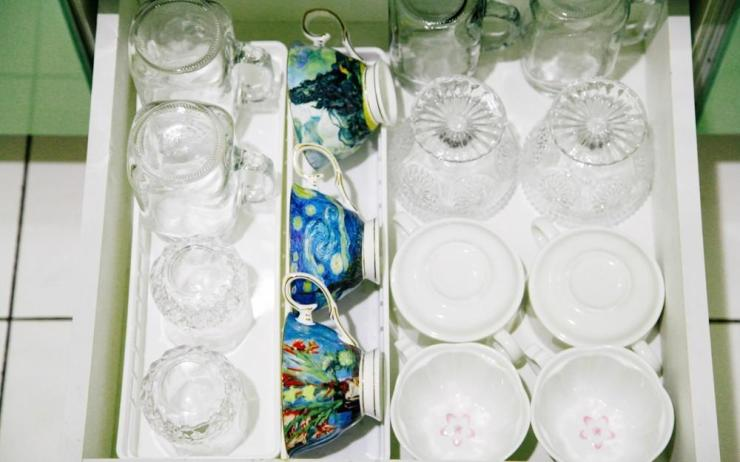厨房的早晨是被香气唤醒的

昨天是椰蓉味

今天是淡奶味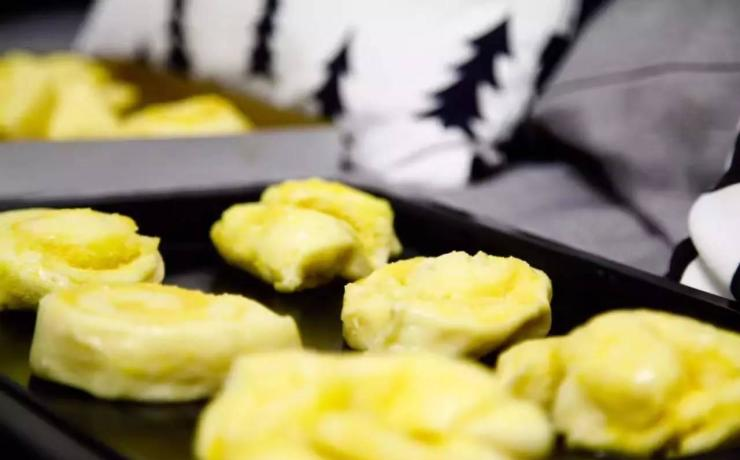春天

新鲜的草莓大批上市

草莓舒芙蕾蛋糕、搭配柠檬黄油小方、

凤梨苹果橙子汁还有草莓酸奶

空气里都冒着粉红色的香甜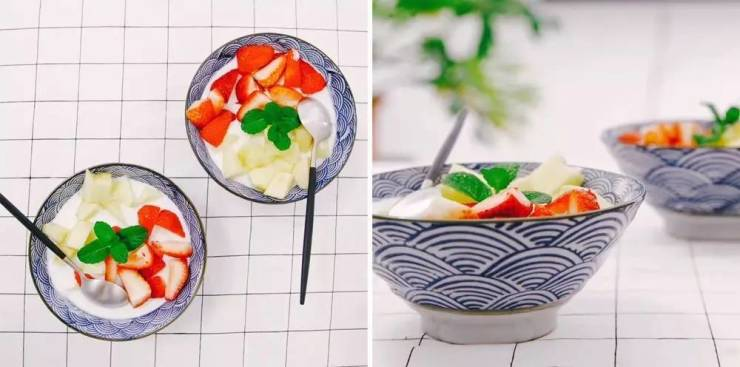夏天

洋梨夏洛特是悠凉的惊喜

红豆抹茶小卷是清新的浪漫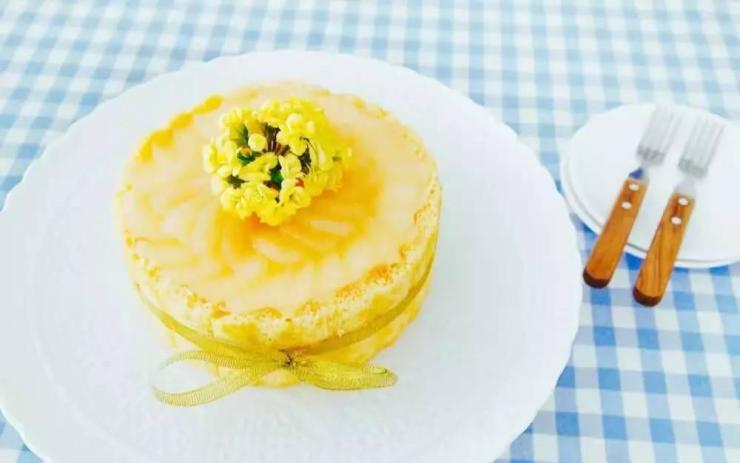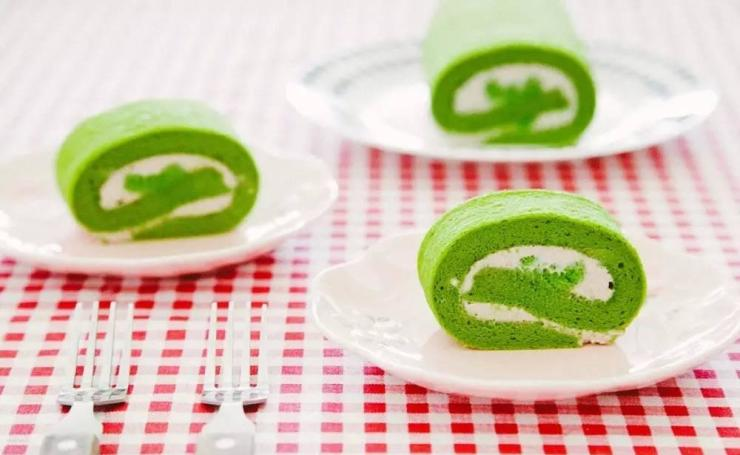秋天

是坚果芝士花棒的颜色

也是香蕉杯子蛋糕的颜色冬天

屋外寒风凛冽

屋内一顿暖烘烘的火锅

盛开了一年里最热闹的缤纷在微博上看到这么一句话：

“不要只把家当做睡觉的地方

那是对人生的敷衍”

那些爱生活的人

最爱的也许就是自己的家对于偌大的北京城来说

想想和大叔

也许都只是匆匆过客

而对于这间50平米的小屋来说

你，装点了我的梦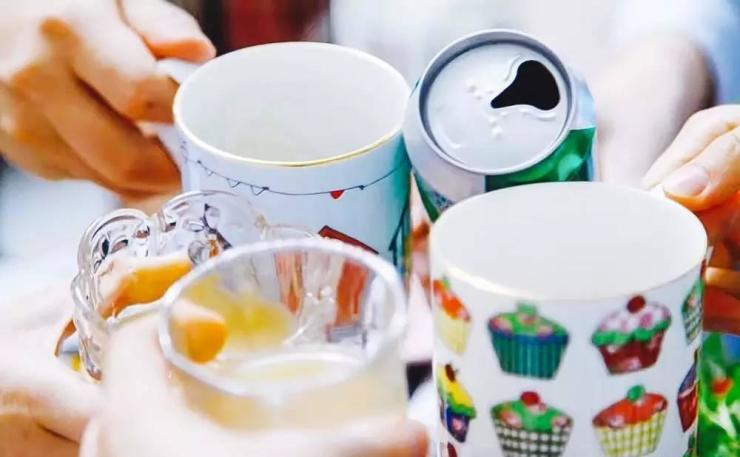`声明：本文由入驻焦点开放平台的作者撰写，除焦点官方账号外，观点仅代表作者本人，不代表焦点立场错误信息举报电话： 400-099-0099，邮箱：jubao@vip.sohu.com，或点此进行意见反馈，或点此进行举报投诉。`A B C D E F G H J K L M N P Q R S T W X Y Z
A - B - C - D - E
• A
• 鞍山
• 安庆
• 安阳
• 安顺
• 安康
• 澳门
• B
• 北京
• 保定
• 包头
• 巴彦淖尔
• 本溪
• 蚌埠
• 亳州
• 滨州
• 北海
• 百色
• 巴中
• 毕节
• 保山
• 宝鸡
• 白银
• 巴州
• C
• 承德
• 沧州
• 长治
• 赤峰
• 朝阳
• 长春
• 常州
• 滁州
• 池州
• 长沙
• 常德
• 郴州
• 潮州
• 崇左
• 重庆
• 成都
• 楚雄
• 昌都
• 慈溪
• 常熟
• D
• 大同
• 大连
• 丹东
• 大庆
• 东营
• 德州
• 东莞
• 德阳
• 达州
• 大理
• 德宏
• 定西
• 儋州
• 东平
• E
• 鄂尔多斯
• 鄂州
• 恩施
F - G - H - I - J
• F
• 抚顺
• 阜新
• 阜阳
• 福州
• 抚州
• 佛山
• 防城港
• G
• 赣州
• 广州
• 桂林
• 贵港
• 广元
• 广安
• 贵阳
• 固原
• H
• 邯郸
• 衡水
• 呼和浩特
• 呼伦贝尔
• 葫芦岛
• 哈尔滨
• 黑河
• 淮安
• 杭州
• 湖州
• 合肥
• 淮南
• 淮北
• 黄山
• 菏泽
• 鹤壁
• 黄石
• 黄冈
• 衡阳
• 怀化
• 惠州
• 河源
• 贺州
• 河池
• 海口
• 红河
• 汉中
• 海东
• 怀来
• I
• J
• 晋中
• 锦州
• 吉林
• 鸡西
• 佳木斯
• 嘉兴
• 金华
• 景德镇
• 九江
• 吉安
• 济南
• 济宁
• 焦作
• 荆门
• 荆州
• 江门
• 揭阳
• 金昌
• 酒泉
• 嘉峪关
K - L - M - N - P
• K
• 开封
• 昆明
• 昆山
• L
• 廊坊
• 临汾
• 辽阳
• 连云港
• 丽水
• 六安
• 龙岩
• 莱芜
• 临沂
• 聊城
• 洛阳
• 漯河
• 娄底
• 柳州
• 来宾
• 泸州
• 乐山
• 六盘水
• 丽江
• 临沧
• 拉萨
• 林芝
• 兰州
• 陇南
• M
• 牡丹江
• 马鞍山
• 茂名
• 梅州
• 绵阳
• 眉山
• N
• 南京
• 南通
• 宁波
• 南平
• 宁德
• 南昌
• 南阳
• 南宁
• 内江
• 南充
• P
• 盘锦
• 莆田
• 平顶山
• 濮阳
• 攀枝花
• 普洱
• 平凉
Q - R - S - T - W
• Q
• 秦皇岛
• 齐齐哈尔
• 衢州
• 泉州
• 青岛
• 清远
• 钦州
• 黔南
• 曲靖
• 庆阳
• R
• 日照
• 日喀则
• S
• 石家庄
• 沈阳
• 双鸭山
• 绥化
• 上海
• 苏州
• 宿迁
• 绍兴
• 宿州
• 三明
• 上饶
• 三门峡
• 商丘
• 十堰
• 随州
• 邵阳
• 韶关
• 深圳
• 汕头
• 汕尾
• 三亚
• 三沙
• 遂宁
• 山南
• 商洛
• 石嘴山
• T
• 天津
• 唐山
• 太原
• 通辽
• 铁岭
• 泰州
• 台州
• 铜陵
• 泰安
• 铜仁
• 铜川
• 天水
• 天门
• W
• 乌海
• 乌兰察布
• 无锡
• 温州
• 芜湖
• 潍坊
• 威海
• 武汉
• 梧州
• 渭南
• 武威
• 吴忠
• 乌鲁木齐
X - Y - Z
• X
• 邢台
• 徐州
• 宣城
• 厦门
• 新乡
• 许昌
• 信阳
• 襄阳
• 孝感
• 咸宁
• 湘潭
• 湘西
• 西双版纳
• 西安
• 咸阳
• 西宁
• 仙桃
• 西昌
• Y
• 运城
• 营口
• 盐城
• 扬州
• 鹰潭
• 宜春
• 烟台
• 宜昌
• 岳阳
• 益阳
• 永州
• 阳江
• 云浮
• 玉林
• 宜宾
• 雅安
• 玉溪
• 延安
• 榆林
• 银川
• Z
• 张家口
• 镇江
• 舟山
• 漳州
• 淄博
• 枣庄
• 郑州
• 周口
• 驻马店
• 株洲
• 张家界
• 珠海
• 湛江
• 肇庆
• 中山
• 自贡
• 资阳
• 遵义
• 昭通
• 张掖
• 中卫

1室1厅1厨1卫1阳台

1
2
3
4
5

0
1
2

1

1

0
1
2
3报名成功，资料已提交审核A B C D E F G H J K L M N P Q R S T W X Y Z
A - B - C - D - E
• A
• 鞍山
• 安庆
• 安阳
• 安顺
• 安康
• 澳门
• B
• 北京
• 保定
• 包头
• 巴彦淖尔
• 本溪
• 蚌埠
• 亳州
• 滨州
• 北海
• 百色
• 巴中
• 毕节
• 保山
• 宝鸡
• 白银
• 巴州
• C
• 承德
• 沧州
• 长治
• 赤峰
• 朝阳
• 长春
• 常州
• 滁州
• 池州
• 长沙
• 常德
• 郴州
• 潮州
• 崇左
• 重庆
• 成都
• 楚雄
• 昌都
• 慈溪
• 常熟
• D
• 大同
• 大连
• 丹东
• 大庆
• 东营
• 德州
• 东莞
• 德阳
• 达州
• 大理
• 德宏
• 定西
• 儋州
• 东平
• E
• 鄂尔多斯
• 鄂州
• 恩施
F - G - H - I - J
• F
• 抚顺
• 阜新
• 阜阳
• 福州
• 抚州
• 佛山
• 防城港
• G
• 赣州
• 广州
• 桂林
• 贵港
• 广元
• 广安
• 贵阳
• 固原
• H
• 邯郸
• 衡水
• 呼和浩特
• 呼伦贝尔
• 葫芦岛
• 哈尔滨
• 黑河
• 淮安
• 杭州
• 湖州
• 合肥
• 淮南
• 淮北
• 黄山
• 菏泽
• 鹤壁
• 黄石
• 黄冈
• 衡阳
• 怀化
• 惠州
• 河源
• 贺州
• 河池
• 海口
• 红河
• 汉中
• 海东
• 怀来
• I
• J
• 晋中
• 锦州
• 吉林
• 鸡西
• 佳木斯
• 嘉兴
• 金华
• 景德镇
• 九江
• 吉安
• 济南
• 济宁
• 焦作
• 荆门
• 荆州
• 江门
• 揭阳
• 金昌
• 酒泉
• 嘉峪关
K - L - M - N - P
• K
• 开封
• 昆明
• 昆山
• L
• 廊坊
• 临汾
• 辽阳
• 连云港
• 丽水
• 六安
• 龙岩
• 莱芜
• 临沂
• 聊城
• 洛阳
• 漯河
• 娄底
• 柳州
• 来宾
• 泸州
• 乐山
• 六盘水
• 丽江
• 临沧
• 拉萨
• 林芝
• 兰州
• 陇南
• M
• 牡丹江
• 马鞍山
• 茂名
• 梅州
• 绵阳
• 眉山
• N
• 南京
• 南通
• 宁波
• 南平
• 宁德
• 南昌
• 南阳
• 南宁
• 内江
• 南充
• P
• 盘锦
• 莆田
• 平顶山
• 濮阳
• 攀枝花
• 普洱
• 平凉
Q - R - S - T - W
• Q
• 秦皇岛
• 齐齐哈尔
• 衢州
• 泉州
• 青岛
• 清远
• 钦州
• 黔南
• 曲靖
• 庆阳
• R
• 日照
• 日喀则
• S
• 石家庄
• 沈阳
• 双鸭山
• 绥化
• 上海
• 苏州
• 宿迁
• 绍兴
• 宿州
• 三明
• 上饶
• 三门峡
• 商丘
• 十堰
• 随州
• 邵阳
• 韶关
• 深圳
• 汕头
• 汕尾
• 三亚
• 三沙
• 遂宁
• 山南
• 商洛
• 石嘴山
• T
• 天津
• 唐山
• 太原
• 通辽
• 铁岭
• 泰州
• 台州
• 铜陵
• 泰安
• 铜仁
• 铜川
• 天水
• 天门
• W
• 乌海
• 乌兰察布
• 无锡
• 温州
• 芜湖
• 潍坊
• 威海
• 武汉
• 梧州
• 渭南
• 武威
• 吴忠
• 乌鲁木齐
X - Y - Z
• X
• 邢台
• 徐州
• 宣城
• 厦门
• 新乡
• 许昌
• 信阳
• 襄阳
• 孝感
• 咸宁
• 湘潭
• 湘西
• 西双版纳
• 西安
• 咸阳
• 西宁
• 仙桃
• 西昌
• Y
• 运城
• 营口
• 盐城
• 扬州
• 鹰潭
• 宜春
• 烟台
• 宜昌
• 岳阳
• 益阳
• 永州
• 阳江
• 云浮
• 玉林
• 宜宾
• 雅安
• 玉溪
• 延安
• 榆林
• 银川
• Z
• 张家口
• 镇江
• 舟山
• 漳州
• 淄博
• 枣庄
• 郑州
• 周口
• 驻马店
• 株洲
• 张家界
• 珠海
• 湛江
• 肇庆
• 中山
• 自贡
• 资阳
• 遵义
• 昭通
• 张掖
• 中卫• 手机• 分享
• 设计
免费设计
• 计算器
装修计算器
• 入驻
合作入驻
• 联系
联系我们
• 置顶
返回顶部